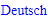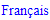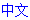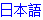# X-Wing

An X-Wing is a fish pattern involving 2 rows and 2 columns.

It is a single-digit solving technique that allows the player to eliminate candidates.

### How it works

When all candidates for a particular digit in 2 rows are located in only 2 columns, completing these rows will also complete these columns. As a result, you may eliminate all candidates for this particular digit from these columns which are not located in the defining rows.

Sounds complicated? Yes. This is a technique that is easier to understand with an example. Take the following fish diagram: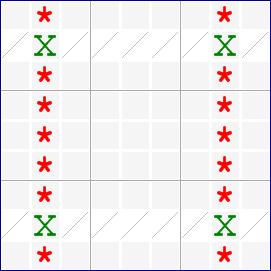There are 2 remaining candidates in row 2 and in row 8, marked X. Both candidates are located in columns 2 and 8. There are only two ways to complete these 2 rows. In both cases, columns 2 and 8 will each be covered. The remaining candidates in these 2 columns can be eliminated. They have been marked with a red asterisk.

### Example

Unfortunately, real puzzle situations do not look as neat as the fish diagram. The grey areas can contain any number of candidates. Here is an example with several additional candidates: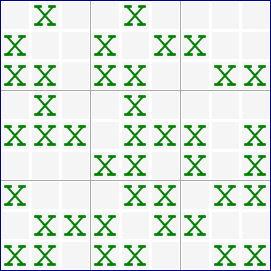You first need to find the X-Wing. In this example, you can find one in rows 1 and 4.Make sure that both rows contain only two candidates each and that these candidates are confined to two columns. In this example, the columns are 2 and 5.

Now we can eliminate the remaining candidates from these two columns.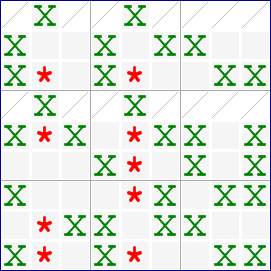A total of 9 candidates can be eliminated from these two columns.

### Notes

An X-Wing can also be found in the columns, causing eliminations in the rows. Turn the example 90 degrees to see how that works.

Neither the rows nor the columns can be located in a single chute. This would cause the pattern to disperse with a few Locked Candidates moves.

﻿

## Sudoku strategies

﻿## Printable Sudoku